Question

If we have C3H8 + 5O2 ---> 3CO2 + 4H2O

a) How many liters of oxygen gas are required to react with 7.2 L of C3H8 if both gasses are at STP?

b) How many grams of CO2 will be produced from 35 L C3H8 at 15 C and 1.65 atm?

c) How many grams of water vapor can be produced when 15 L C3H8 at 15 C and 1.65 atm are reacted with 15 L O2 at -20.0 C and 19.2 psi?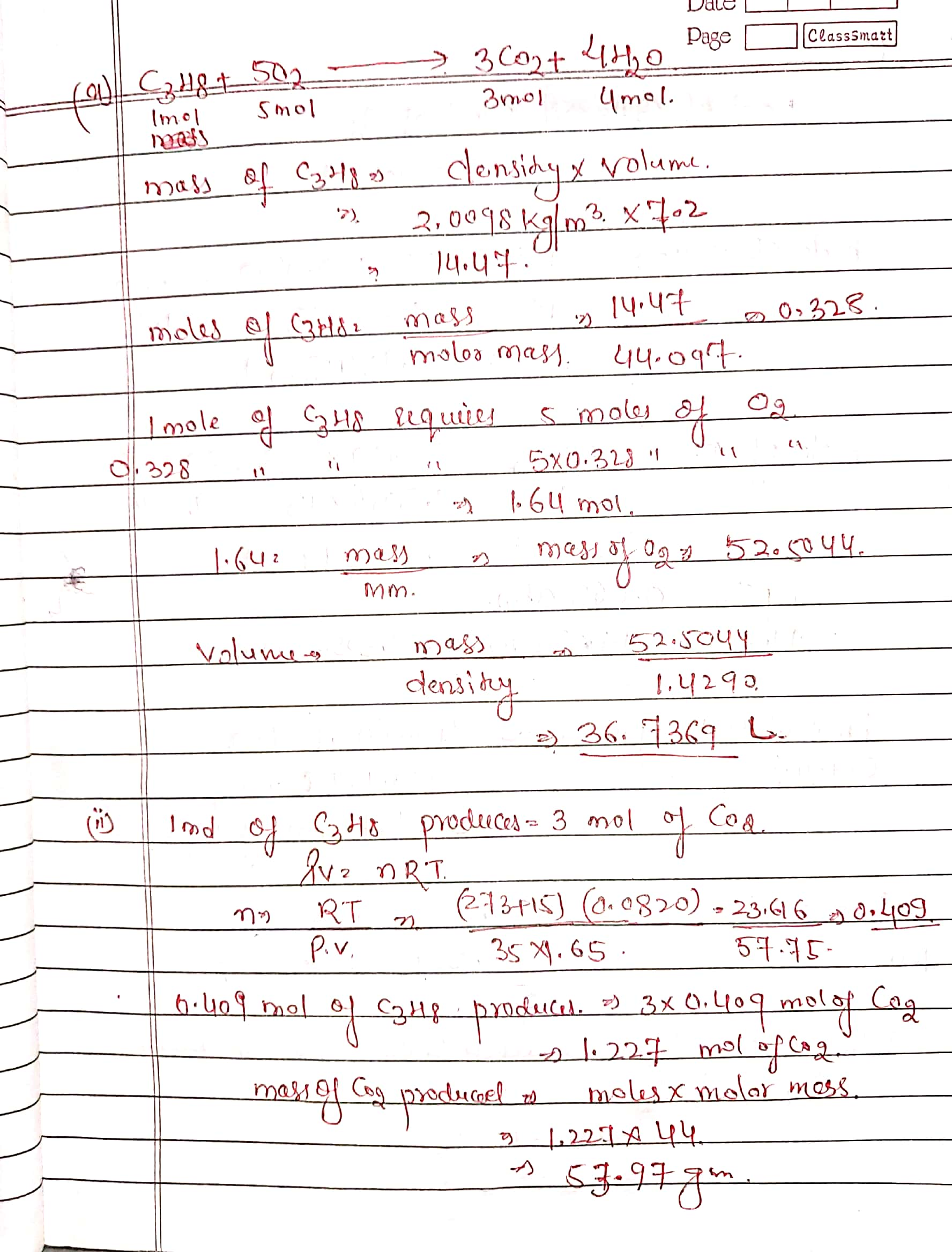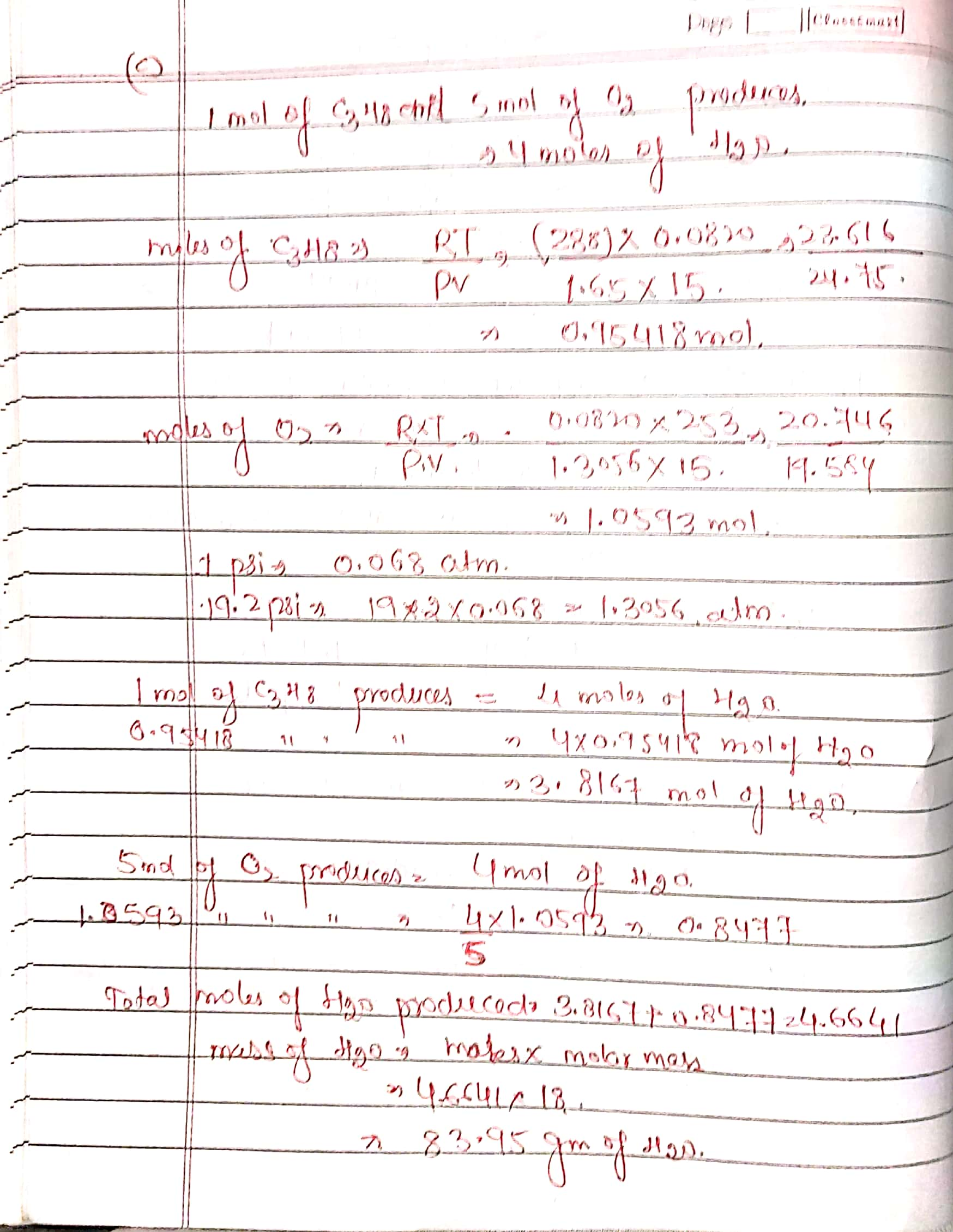#### Earn Coins

Coins can be redeemed for fabulous gifts.

Similar Homework Help Questions
• ### Please help? Calculate deltaHr for the reaction: C3H8(g) + 5O2(g)----->3CO2(g) + 4H2O(l) Given: 3C(s) + 4H2(g)----->C3H8(g)...

Please help? Calculate deltaHr for the reaction: C3H8(g) + 5O2(g)----->3CO2(g) + 4H2O(l) Given: 3C(s) + 4H2(g)----->C3H8(g) deltaH -24.8 kcal    H2(g) + 1/2 O2(g)----->H2O(l) deltaH -68.3kcal C(s) + O2(g)-------> CO2(g) deltaH -94.0 kcal

• ### How many grams of O2(g) are needed to completely burn 35.9 g of C3H8(g)? C3H8 +...

How many grams of O2(g) are needed to completely burn 35.9 g of C3H8(g)? C3H8 + 5O2 ---> 3CO2 + 4H2O

• ### The balanced chemical equation for the combustion of propane is C3H8(g) + 5O2(g) +3CO2(g) + 4H2O(g)...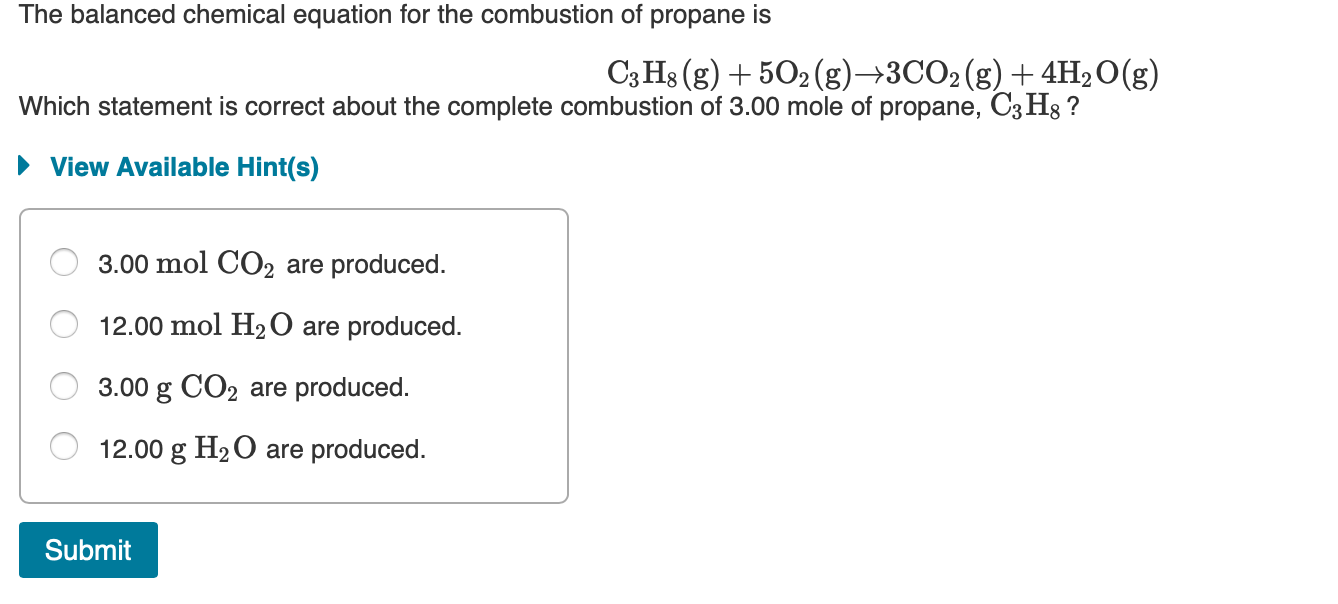The balanced chemical equation for the combustion of propane is C3H8(g) + 5O2(g) +3CO2(g) + 4H2O(g) Which statement is correct about the complete combustion of 3.00 mole of propane, C3Hg ? ► View Available Hint(s) O 3.00 mol CO2 are produced. O 12.00 mol H2O are produced. O 3.00 g CO2 are produced. O 12.00 g H2O are produced. Submit

• ### The propane fuel (C3H8) used in gas barbeques burns according to this thermochemical equation. C3H8(g)+5O2(g)→3CO2(g)+4H2O(g)ΔH∘rxn=−2044kJ If...

The propane fuel (C3H8) used in gas barbeques burns according to this thermochemical equation. C3H8(g)+5O2(g)→3CO2(g)+4H2O(g)ΔH∘rxn=−2044kJ If a pork roast must absorb 1.7×103 kJ to fully cook, and if only 15 % of the heat produced by the barbeque is actually absorbed by the roast, what mass of CO2 is emitted into the atmosphere during the grilling of the pork roast?

• ### The propane fuel (C3H8) used in gas barbecues burns according to the following equation: C3H8(g)+5O2(g)→3CO2(g)+4H2O(g)ΔH∘rxn=−2044kJ PART...

The propane fuel (C3H8) used in gas barbecues burns according to the following equation: C3H8(g)+5O2(g)→3CO2(g)+4H2O(g)ΔH∘rxn=−2044kJ PART A: If a pork roast must absorb 2.3×103kJ to fully cook, and if only 10.% of the heat produced by the barbecue is actually absorbed by the roast, what mass of CO2 is emitted into the atmosphere during the grilling of the pork roast?

• ### Calculate the amount reacted or produced in each of the following equations and state which gas law you used to solve the problem1) 2K(s) +CL2(g)--> KCL(s) How many liters of Cl2 gas at STP are required to completely react 8.50 g K

Calculate the amount reacted or produced in each of the following equations and state which gas law you used to solve the problem1) 2K(s) +CL2(g)--> KCL(s) How many liters of Cl2 gas at STP are required to completely react 8.50 g K?2) 2KClO3(s)--> 2KCL(s) + 3O2(g) How many grams KClO3 must be heated to produce 12.6 L O2 gas at STP?3) 4NH3(g) + 5O2(g)-->4NO(g) + 6H2O(l) How many liters NO gas at STP can be produced when 55.8 L O2...

• ### If 2.72 mol of propane are reacted with excess oxygen according to C3H8 +502 3CO2 + 4H20 how many grams of H20 are p...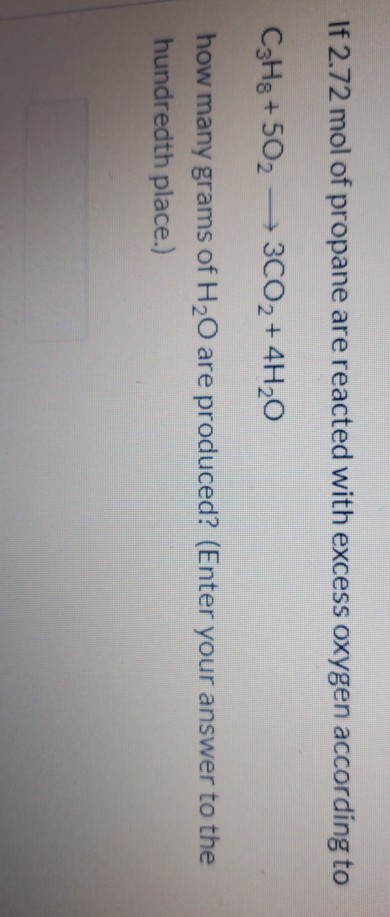If 2.72 mol of propane are reacted with excess oxygen according to C3H8 +502 3CO2 + 4H20 how many grams of H20 are produced? (Enter your answer to the hundredth place.)

• ### If 2.45 mol of propane are reacted with excess oxygen according to C3H8 +502 — 3CO2...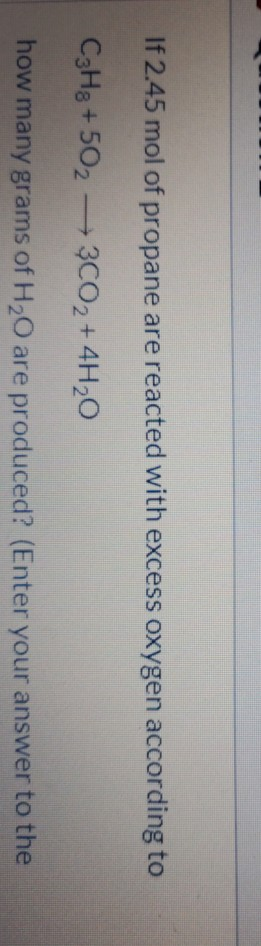If 2.45 mol of propane are reacted with excess oxygen according to C3H8 +502 — 3CO2 + 4H20 how many grams of H20 are produced? (Enter your answer to the

• ### How many liters of oxygen at 23° C and 1.12 atm will be required for the...

How many liters of oxygen at 23° C and 1.12 atm will be required for the combustion of 10.0 g of methane, CH4, according to the chemical reaction below:                                  CH4 (g) + 2 O2 (g)­ ---> CO2 (g) + 2 H2O (g)   When magnesium burns in air, the reaction is described by:  2 Mg (s) + O2 (g) ---> 2 MgO (s) What mass of magnesium will react with 10.8 L of oxygen if the temperature and pressure of the...

• ### 2C2H2+5O2=4CO2+2H2O If one starts with 4 moles of O2, how many moles of C2H2 will react...

2C2H2+5O2=4CO2+2H2O If one starts with 4 moles of O2, how many moles of C2H2 will react with it? How many moles of CO2 will be produced? How many moles of H2O will be produced? Starting with 15 moles of C2H2, how many moles of O2 are required to react with it? How many moles of CO2 will be produced? How many moles of H2O will be produced? Starting with 130g of C2H2, how many grams of O2 are required? How...